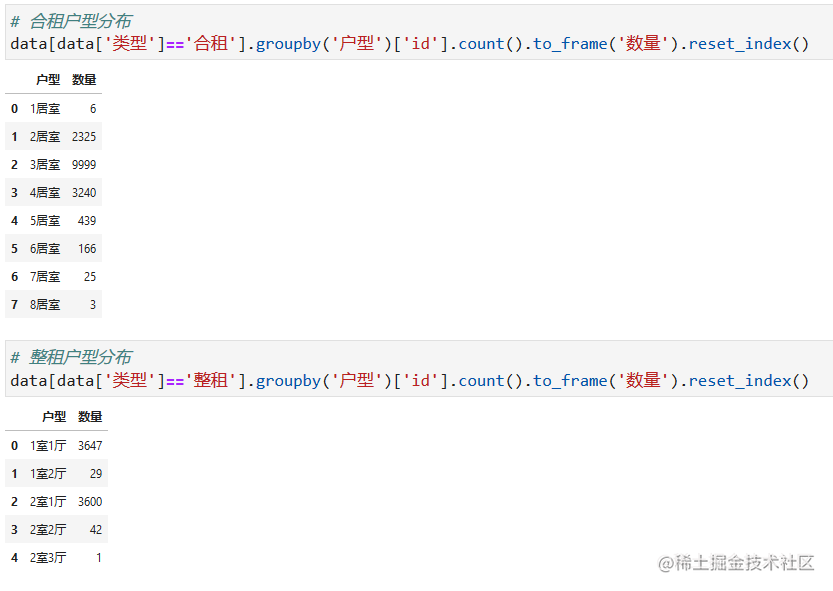# Python爬取北京2.3万条租房信息，发现快租不起房子了！

## 1.概述

PS：如有需要Python学习资料的小伙伴可以加点击下方链接自行获取

## 2.数据采集-爬虫

### 2.1.房屋信息解析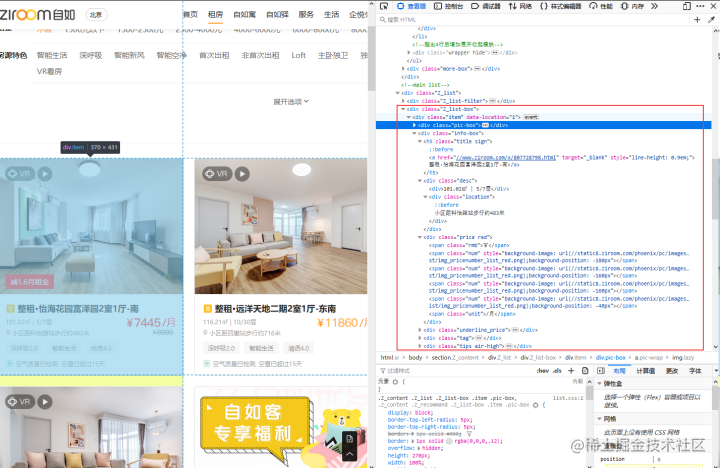``````# 获取具体房屋信息
houseId = re.findall('x/(.*?).html"target="_blank">', item)
title = re.findall('target="_blank">(.*?)</a>',item) # 房屋信息 朝向-小区-户型-卧次
large = re.findall('<div>(.*?)</div>', item) # 房屋 面积-楼层
location = re.findall('<divclass="location">(.*?)</div>', item) # 房屋 面积-楼层

### 2.2.房屋价格解析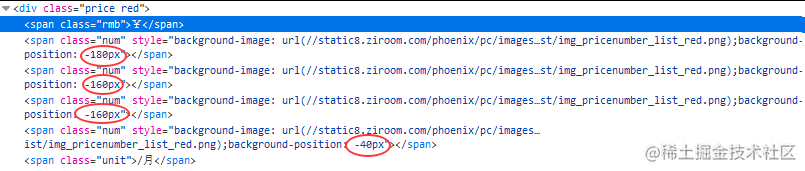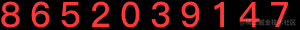``````backgroundHtml = re.findall('url\((.*?)\)', item)
priceList = re.findall('background-position:(.*?)px"></span>', item)# 房价数字图片地址

image = requests.get('http:' + backgroundHtml).content
# 保存价格用图片到本地
f = open('.\price.png', 'wb')
f.write(image)
f.close()
# 调用函数（见下面函数定义）获取价格数字字符串
text = get_pricetext()

# 获取价格
price = ''
for i in priceList:
num = int(float(i) / -20) #对于不同情况中有折扣的是20，无折扣的是21.4
price = price + text[num]

``````# 由于数字图是png无法被识别，所以我们需要进行背景加白
def get_pricetext():
# 给透明图片加白色背景
im = Image.open('.\price.png')
x,y = im.size
try:
p = Image.new('RGBA', im.size, (255,255,255))
p.paste(im, (0, 0, x, y), im)
p.save('.\price.png')
except:
pass

# 获取图片中数字字符串
text = pytesseract.image_to_string(Image.open(".\price.png"),
config='--psm 10 --oem 3 -c tessedit_char_whitelist=1234567890',
lang='eng')
text = re.sub('\s','',text)

return text

## 3.数据处理-清洗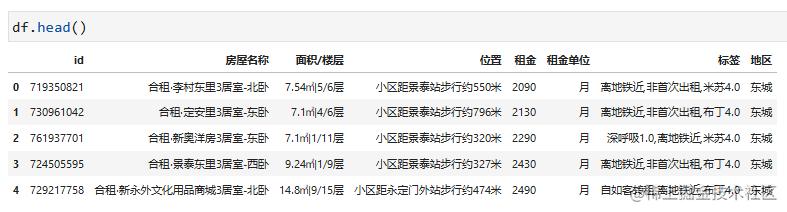### 3.1.房屋名称数据清洗

``````# 房屋信息解析
# s = '合租·李村东里3居室-北卧'
# s = '合租·强佑·府学上院4居室-北卧'
# s = '整租·铁二区1室1厅-北'
# s = '整租·厂甸11号院1室1厅-东'
s = '整租·牛街182室1厅-西'
re.split(r'(.*?)·(.*)(\d居*室.*)-(.*)',s)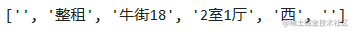``````df['类型'] = df['房屋名称'].apply(lambda x : re.split(r'(.*?)·(.*)(\d居*室.*)-(.*)',x))
df['小区'] = df['房屋名称'].apply(lambda x : re.split(r'(.*?)·(.*)(\d居*室.*)-(.*)',x))
df['户型'] = df['房屋名称'].apply(lambda x : re.split(r'(.*?)·(.*)(\d居*室.*)-(.*)',x))
df['卧室朝向'] = df['房屋名称'].apply(lambda x : re.split(r'(.*?)·(.*)(\d居*室.*)-(.*)',x))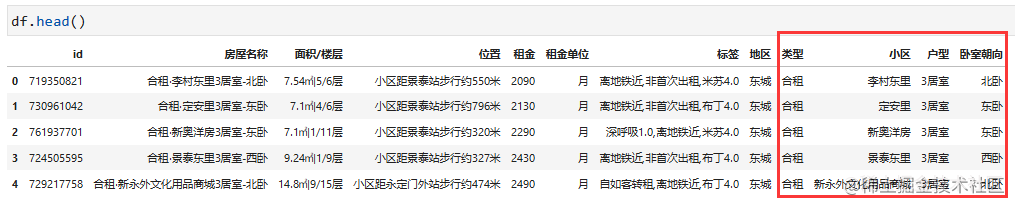### 3.2.房间信息数据清洗

``````# 房间信息解析
# 我们在数据处理中发现存在异常数据（楼层如 7层 或 -1/5层）
s = '87.26㎡|11/29层'
re.split(r'(.*?)㎡\|(-?\d+)\/?(.*?)层',s)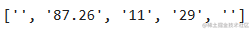``````df['房间大小'] = df['面积/楼层'].apply(lambda x : re.split(r'(.*?)㎡\|(-?\d+)\/?(.*?)层',x))
df['房间楼层'] = df['面积/楼层'].apply(lambda x : re.split(r'(.*?)㎡\|(-?\d+)\/?(.*?)层',x))
df['房间楼房层数'] = df['面积/楼层'].apply(lambda x : re.split(r'(.*?)㎡\|(-?\d+)\/?(.*?)层',x))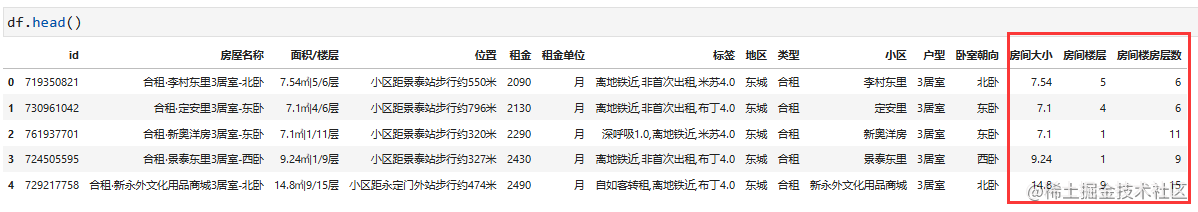### 3.3.位置信息数据清洗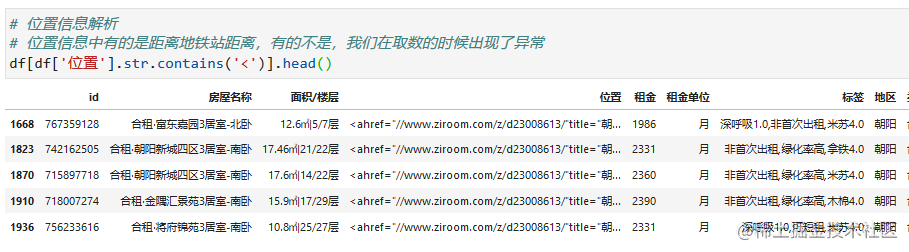``````# 替换非地铁信息位置
df['位置'] = df['位置'].apply(lambda x: re.sub('<(.*?)>','',x))
# 获取最近地铁站
def getMetro(x):
if len(x) >= 9:
return re.split(r'小区距(.*?)步行约(\d+?)米',x)
else:
return ''
# 获取距离地铁站的距离
def getDistance(x):
if len(x) >= 9:
return re.split(r'小区距(.*?)步行约(\d+?)米',x)
else:
return ''

df['附近地铁站'] = df['位置'].apply(getMetro)
df['距离地铁站距离'] = df['位置'].apply(getDistance)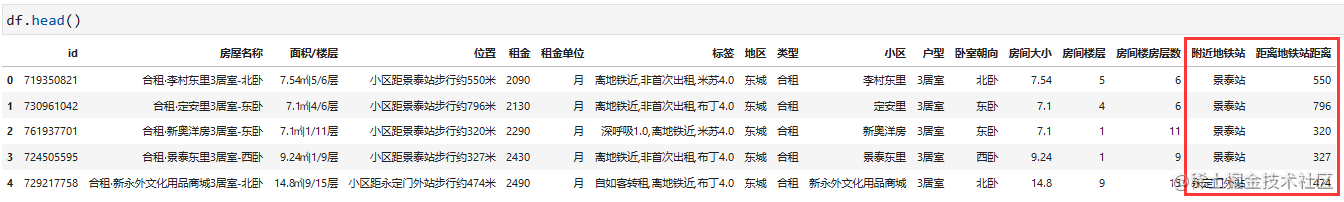### 3.4.选取需要用于分析的字段

``````data = df[['id', '房屋名称', '租金', '租金单位', '标签', '地区', '类型', '小区', '户型',
'卧室朝向', '房间大小', '房间楼层', '房间楼房层数', '附近地铁站', '距离地铁站距离']]
# 计算 租金 元/月/平米，取小数点后2位
data.loc[data['租金单位']=='月', 'price'] = round(data['租金']/data['房间大小'].astype(float),2)
data.loc[data['租金单位']=='天', 'price'] = round(30*data['租金']/data['房间大小'].astype(float),2)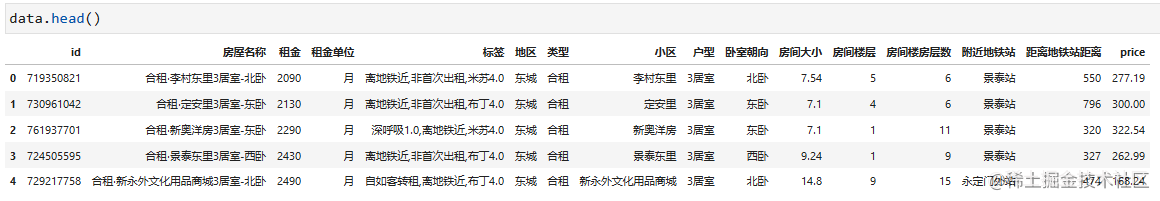## 4.房源相关数据统计与可视化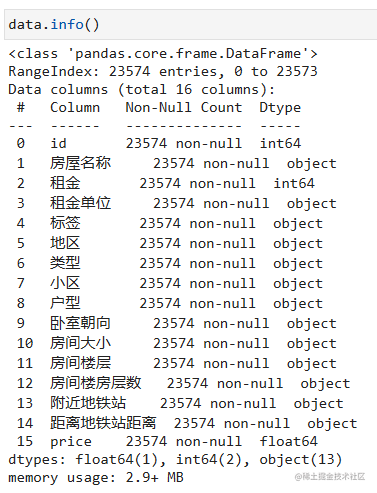``````# 引入需要用到的库
from pyecharts.globals import CurrentConfig, NotebookType
CurrentConfig.NOTEBOOK_TYPE = NotebookType.JUPYTER_LAB
from pyecharts import options as opts
from pyecharts.commons.utils import JsCode
from pyecharts.charts import *

### 4.1.房源分布(map)

``````# 统计各地区房源数
beijing = data.groupby('地区',as_index=False)['id'].count().sort_values('id',ascending=False).reset_index(drop=True)
beijing.loc[~beijing['地区'].str.endswith('区'),'地区']=beijing['地区']+'区'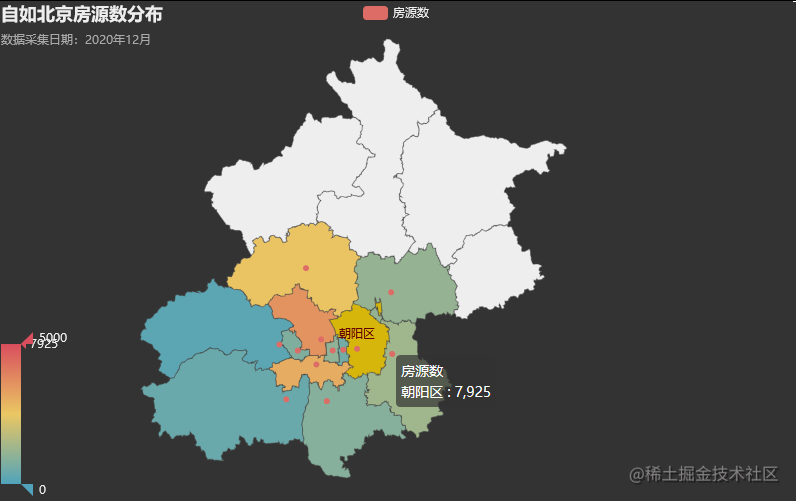``````# 绘制地图（区）
c = (
Map(init_opts=opts.InitOpts(theme='dark', width='800px'))
.add("房源数", [list(z) for z in zip(beijing['地区'].to_list(), beijing['id'].to_list())], "北京", label_opts=opts.LabelOpts(is_show=False))
.set_global_opts(
title_opts=opts.TitleOpts(title="自如北京房源数分布",
subtitle='数据采集日期：2020年12月'),
visualmap_opts=opts.VisualMapOpts(max_=5000)
)
)
# c.render('map_beijing.html')
c.render_notebook()

### 4.2.各地区房源数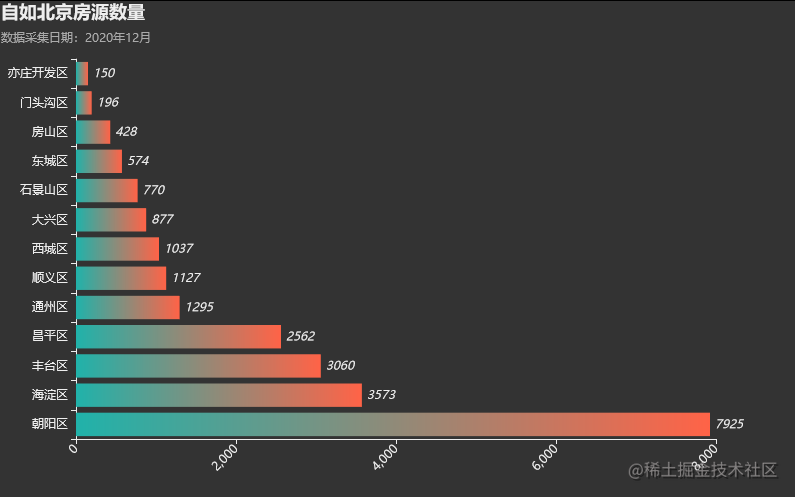``````# 绘制柱状图
bar = (Bar(init_opts=opts.InitOpts(theme='dark', width='800px'))
.set_series_opts(label_opts=opts.LabelOpts(is_show=True,
position='right',
font_style='italic'),
itemstyle_opts=opts.ItemStyleOpts(
[{
offset: 0,
color: 'rgb(255,99,71)'
}, {
offset: 1,
color: 'rgb(32,178,170)'
}])"""))
)
.set_global_opts(
title_opts=opts.TitleOpts(title="自如北京房源数量",
subtitle='数据采集日期：2020年12月'),
xaxis_opts=opts.AxisOpts(axislabel_opts=opts.LabelOpts(rotate=45)),
legend_opts=opts.LegendOpts(is_show=True))
.reversal_axis()
)
bar.render_notebook()

### 4.3.各区房源数占比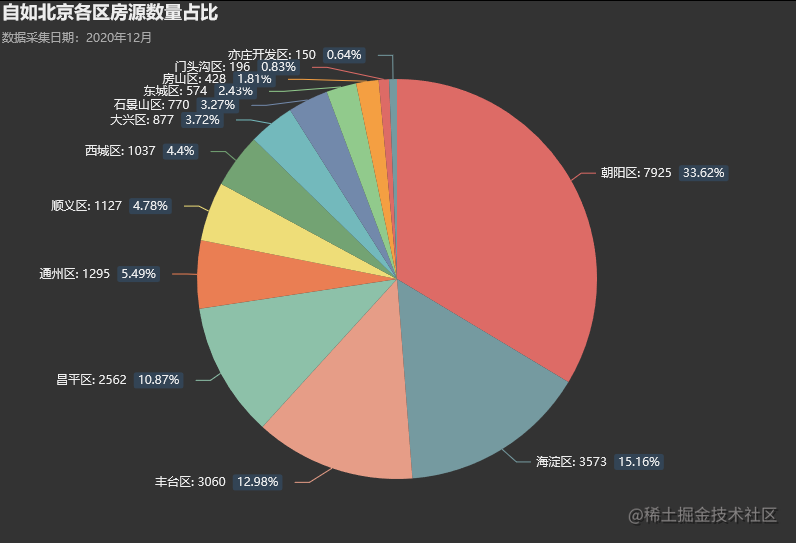``````# 富文本
rich_text = {
"a": {"color": "#999", "lineHeight": 22, "align": "center"},
"b": {"fontSize": 12, "lineHeight": 33},
"per": {
"color": "#eee",
"backgroundColor": "#334455",
},
}

location = beijing['地区'].to_list()
num = beijing['id'].to_list()

pie = (Pie(init_opts=opts.InitOpts(theme='dark', width='800px',
height='560px',
))
.add('房源数', [list(z) for z in zip(location, num)],
label_opts=opts.LabelOpts(position='outsiede',
formatter="{b|{b}: }{c}  {per|{d}%}  ",
rich=rich_text))
.set_global_opts(legend_opts=opts.LegendOpts(is_show=False),
title_opts=opts.TitleOpts(title="自如北京各区房源数量占比",
subtitle='数据采集日期：2020年12月'),)
)
pie.render_notebook()

### 4.4.租房类型分布

``````# 租房类型分布统计
houseType = data.pivot_table(values='id',index='地区',columns='类型',aggfunc='count').fillna('')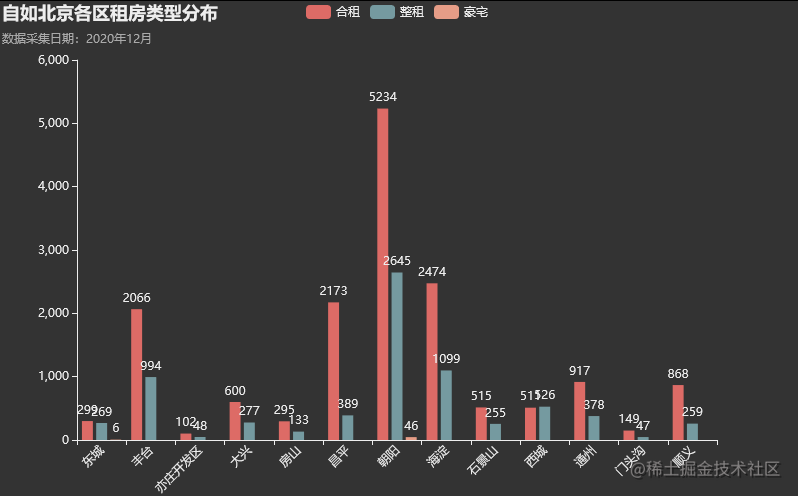``````# 绘制租房类型分布
bar = (
Bar(init_opts=opts.InitOpts(theme='dark', width='800px'))
.set_global_opts(legend_opts=opts.LegendOpts(is_show=True),
xaxis_opts=opts.AxisOpts(axislabel_opts=opts.LabelOpts(rotate=45)),
title_opts=opts.TitleOpts(title="自如北京各区租房类型分布",
subtitle='数据采集日期：2020年12月'),
)
)
bar.render_notebook()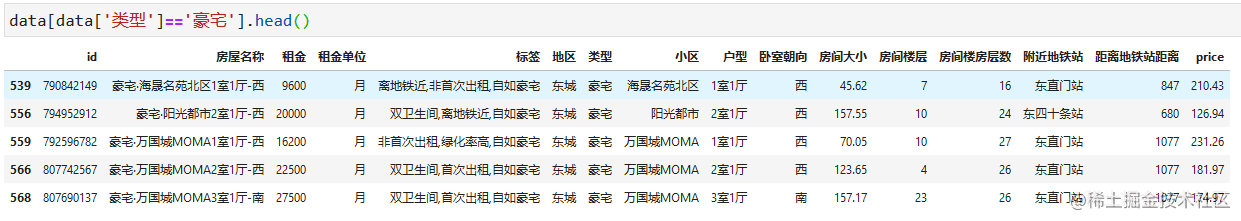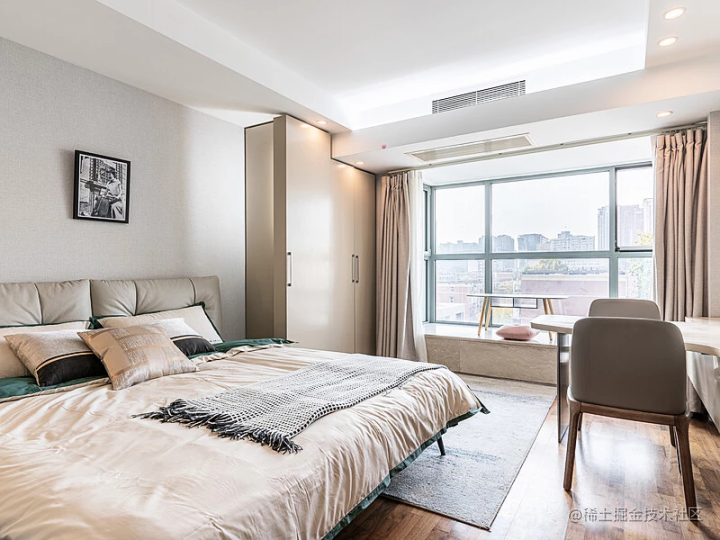### 4.5.地铁站附近房源数据

``````metro = data.groupby(['地区','附近地铁站'],as_index=False)['id'].count().sort_values(['地区','id'],ascending=False).reset_index(drop=True)
metro = metro[metro['附近地铁站']!='']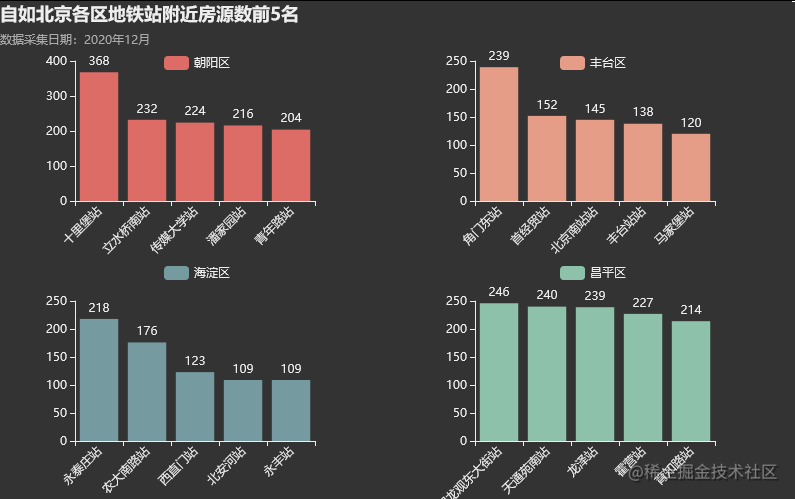``````# 绘制组合图
bar1 = (Bar()
.set_global_opts(legend_opts=opts.LegendOpts(is_show=True, pos_right="70%",pos_top="10%"),
xaxis_opts=opts.AxisOpts(axislabel_opts=opts.LabelOpts(rotate=45)),
title_opts=opts.TitleOpts(title="自如北京各区地铁站附近房源数前5名",
subtitle='数据采集日期：2020年12月'))
)

bar2 = (Bar()
.set_global_opts(legend_opts=opts.LegendOpts(is_show=True, pos_right="70%",pos_top="52%"),
xaxis_opts=opts.AxisOpts(axislabel_opts=opts.LabelOpts(rotate=45)))
)

bar3 = (Bar()
.set_global_opts(legend_opts=opts.LegendOpts(is_show=True, pos_left="70%", pos_top="10%"),
xaxis_opts=opts.AxisOpts(axislabel_opts=opts.LabelOpts(rotate=45)))
)

bar4 = (Bar()
.set_global_opts(legend_opts=opts.LegendOpts(is_show=True, pos_left="70%",pos_top="52%"),
xaxis_opts=opts.AxisOpts(axislabel_opts=opts.LabelOpts(rotate=45)))
)

grid = (Grid(init_opts=opts.InitOpts(theme='dark', width='800px',))

)
grid.render_notebook()

### 4.6.租金前10名地铁站圈

``````metroPrice = data.groupby('附近地铁站',as_index=False)['price'].mean().sort_values('price',ascending=False).reset_index(drop=True)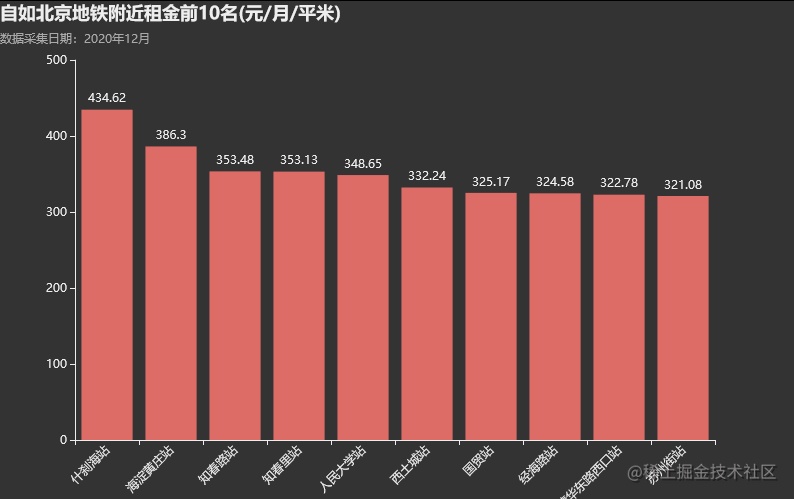``````# 绘制柱状图
bar = (
Bar(init_opts=opts.InitOpts(theme='dark', width='800px'))
.set_series_opts(label_opts=opts.LabelOpts(formatter='{c}'))
.set_global_opts(legend_opts=opts.LegendOpts(is_show=True),
xaxis_opts=opts.AxisOpts(axislabel_opts=opts.LabelOpts(rotate=45)),
title_opts=opts.TitleOpts(title="自如北京地铁附近租金前10名(元/月/平米)",
subtitle='数据采集日期：2020年12月'),
)
)
bar.render_notebook()

### 4.7.各区合租类平均租金箱线图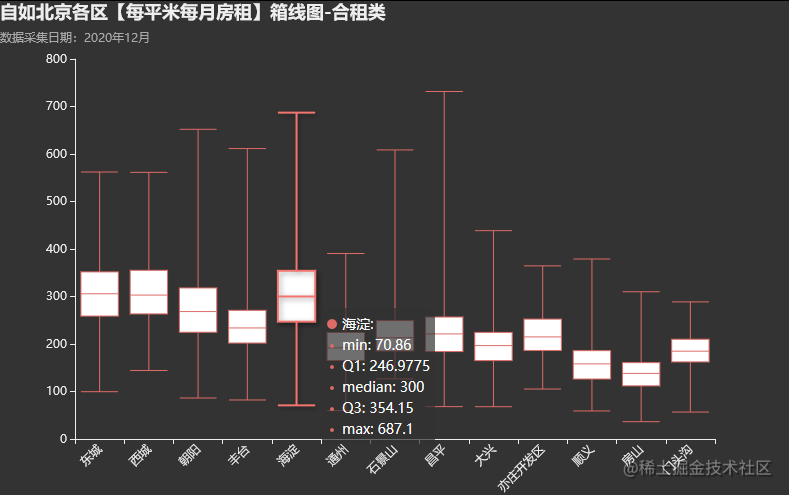``````# 箱线图
# 合租类
x_data = list(data['地区'].unique())
y_data = [data[(data['地区']==x)&(data['类型']=='合租')]['price'].to_list() for x in x_data]

Box = (Boxplot(init_opts=opts.InitOpts(theme='dark', width='800px'))
.set_global_opts(legend_opts=opts.LegendOpts(is_show=True),
xaxis_opts=opts.AxisOpts(axislabel_opts=opts.LabelOpts(rotate=45)),
title_opts=opts.TitleOpts(title="自如北京各区【每平米每月房租】箱线图-合租类",
subtitle='数据采集日期：2020年12月'),
)
)
Box.render_notebook()

### 4.8.各区整租类平均租金箱线图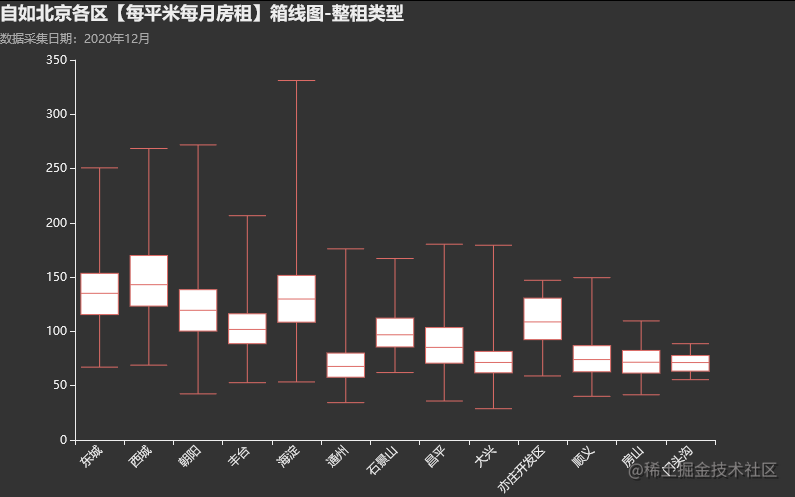## 5.房间相关数据统计与可视化

``````# 引入相关绘图库
import matplotlib.pyplot as plt
plt.rcParams['font.family'] = ['Microsoft YaHei'] #设置全局默认字体 为 幼圆
plt.rcParams['axes.unicode_minus'] = False # 解决中文字体下负号显示问题

import seaborn as sns
sns.set_style("darkgrid",{"font.family":['Microsoft YaHei', 'SimHei']})   #seaborn绘图的字体设置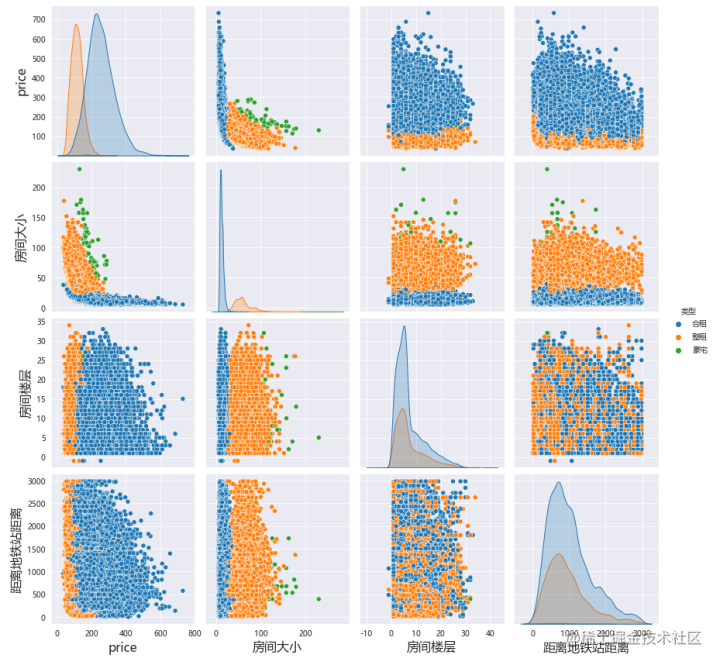``````plt.rcParams["axes.labelsize"] = 16  # 设置全局轴标签字典大小
# 由于部分房源未标注距离地铁站距离，这里我们只选有此数据的
score = data[data['距离地铁站距离']!=''][['price','房间大小','房间楼层','距离地铁站距离','类型']]
score['距离地铁站距离'] = score['距离地铁站距离'].astype('int')
# 散点图矩阵
sns.pairplot(score, hue='类型',height=3)

### 5.1.合租类房间大小直方图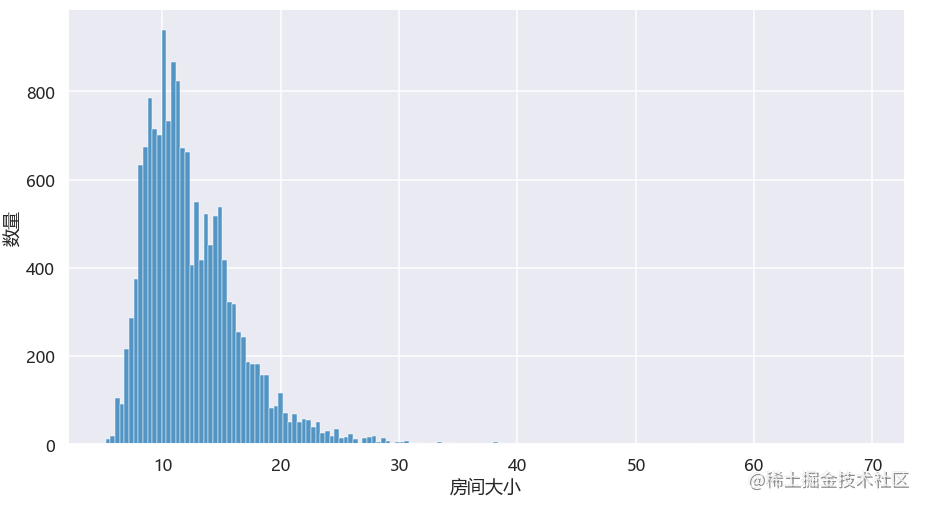``````plt.figure(figsize=(15,8))
sns.set_context("talk")
sns.histplot(data[data['类型']=='合租'].房间大小).set(ylabel='数量')

### 5.2.整租类房间大小直方图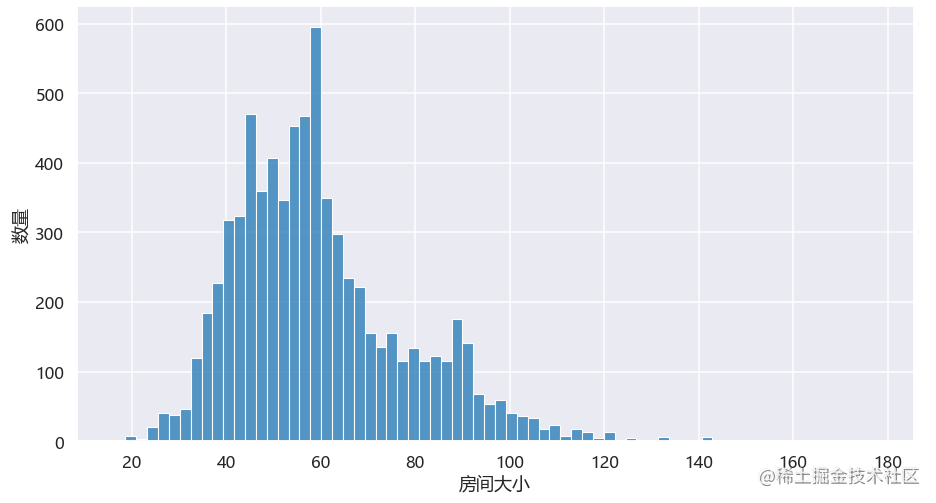### 5.3.合租类房间租金直方图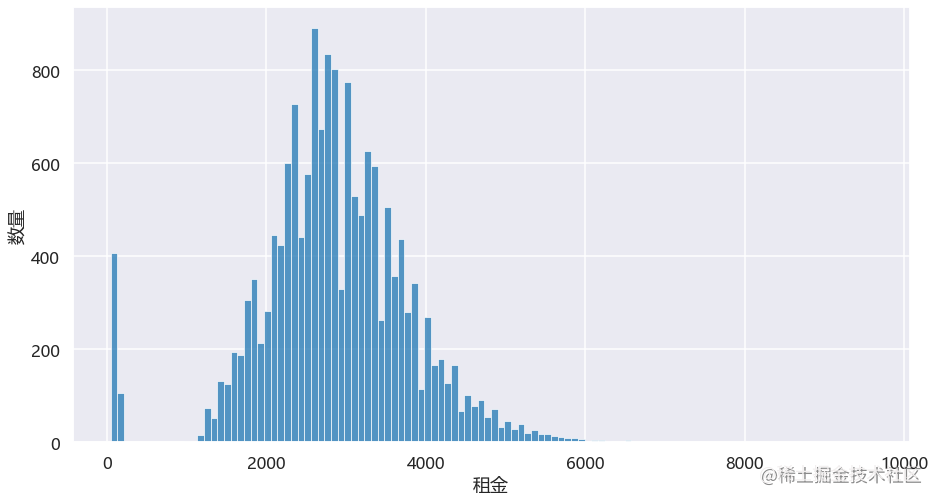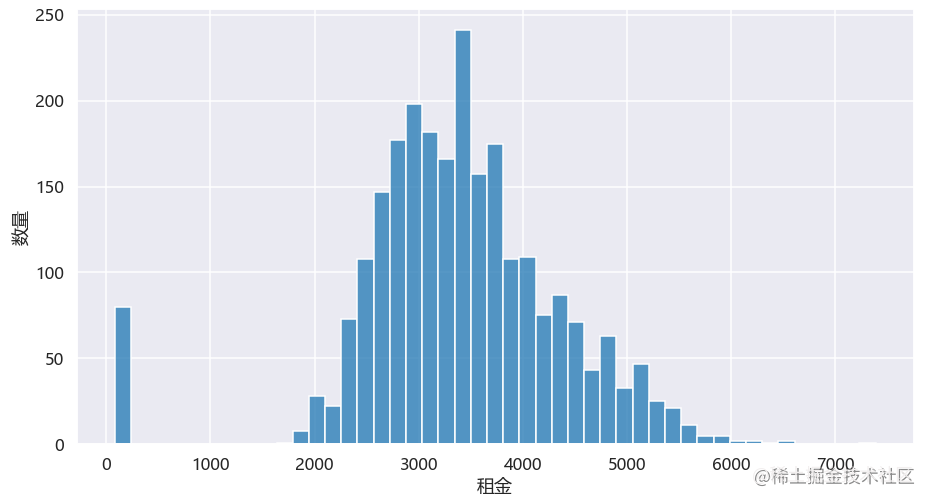### 5.4.整租类房间租金直方图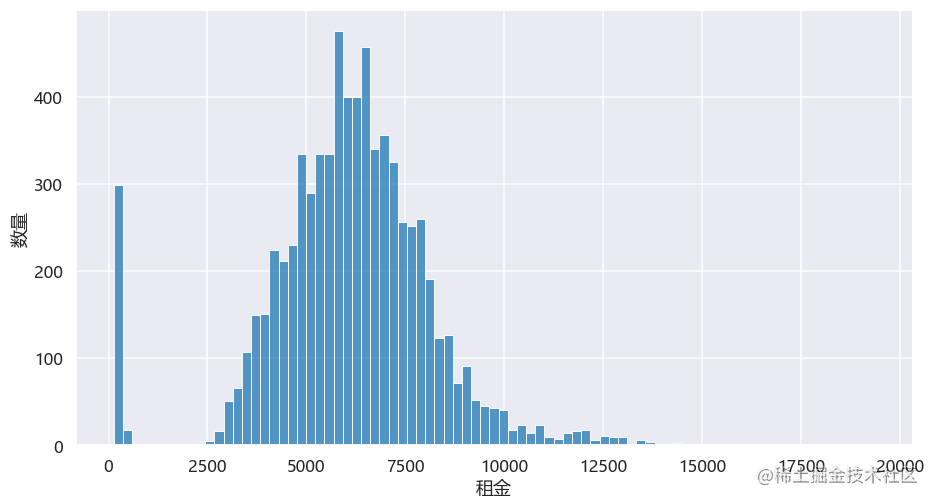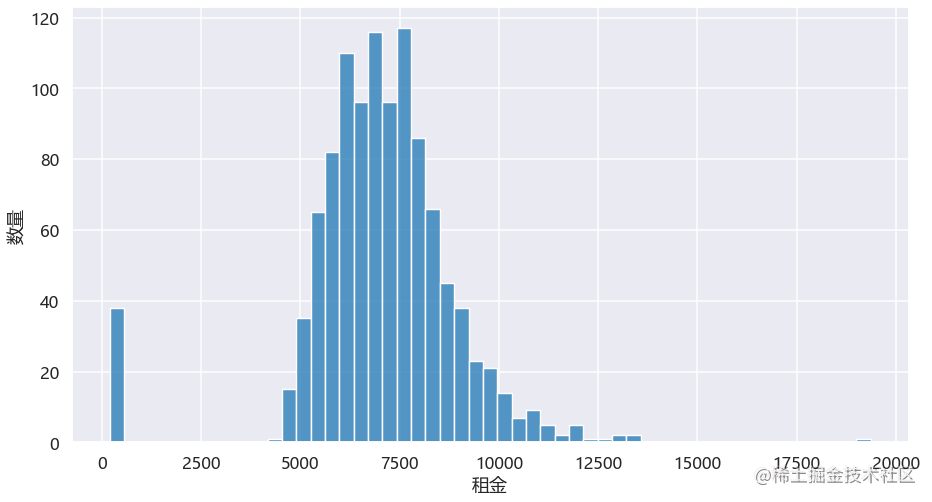### 5.5.房源距离地铁站距离直方图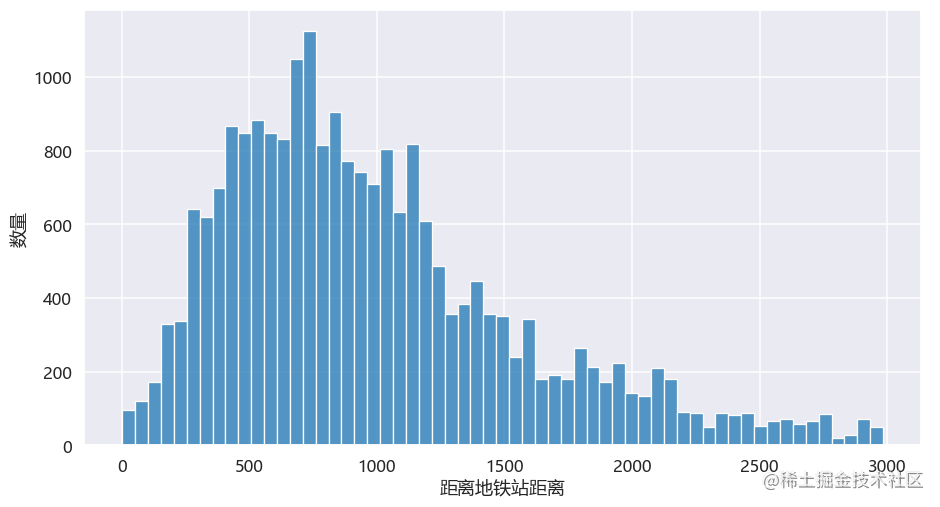### 5.6.平米租金与距离地铁站距离回归图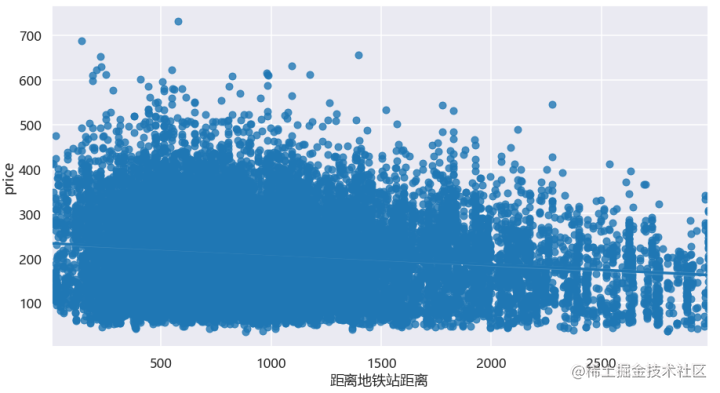### 5.7.各区卧室朝向与平米租金热力图

``````# 数据统计
data_table = data[data['类型']=='合租'].pivot_table(values='price',index='卧室朝向',columns='地区',aggfunc='mean')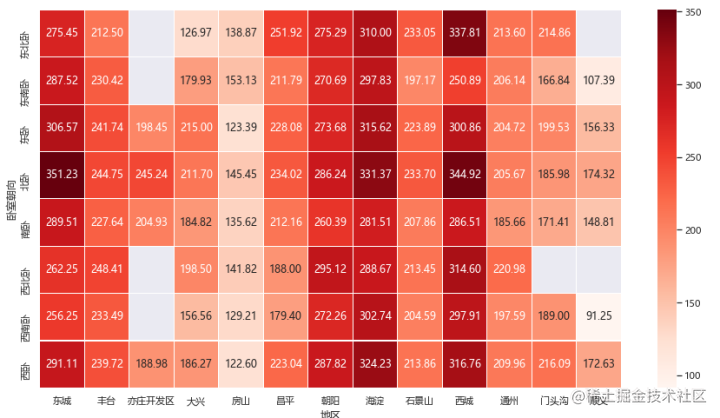``````# 卧室朝向价格热力图
plt.figure(figsize=(15,8))
# sns.set_context("talk")
sns.set_context("notebook")
sns.heatmap( data_table, cmap='Reds', annot=True, fmt='0.2f', linewidths=0.2)

### 5.8.户型分布